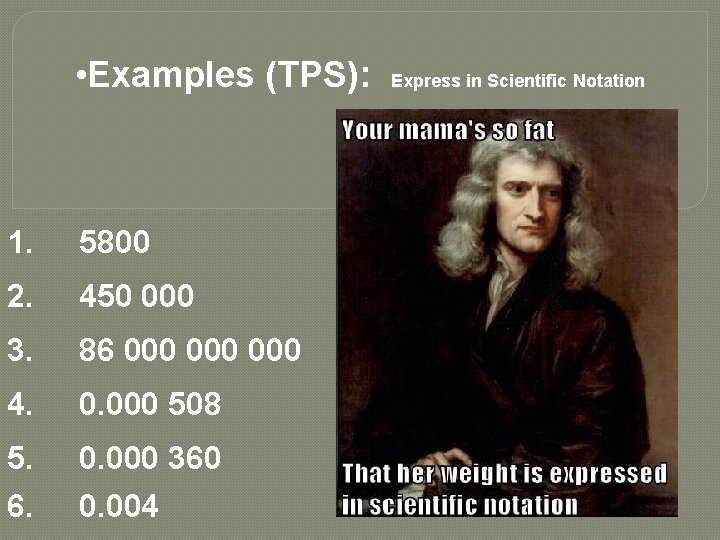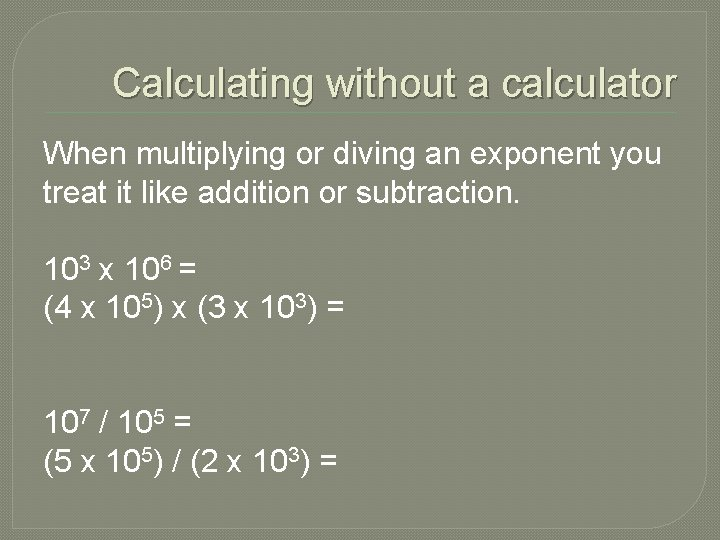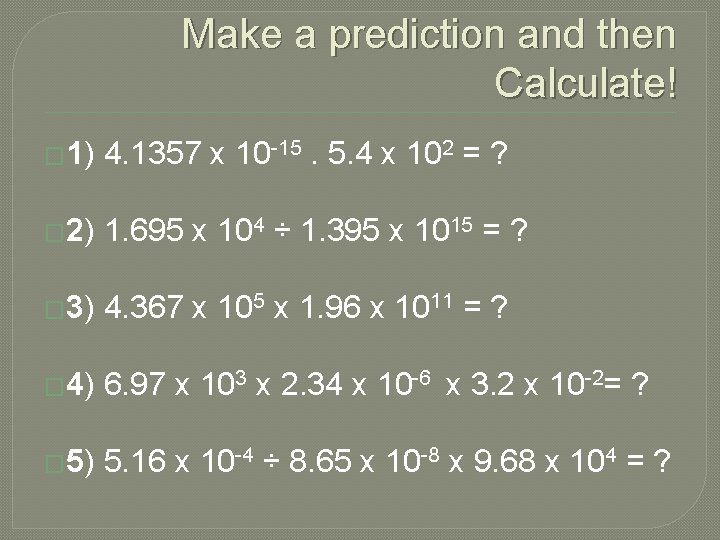# Scientific Notation in Science Convert from Scientific Notation

• Slides: 9Scientific Notation in ScienceConvert from Scientific Notation to Real Number: 5. 14 x 105 = • Scientific notation consists of a coefficient (here 5. 14) multiplied by 10 raised to an exponent (here 5). • If the exponent is a positive number, you move that many spaces to the right. • If the exponent is a negative number, you move that many spaces to the left.• Convert from Real Number to Scientific Notation: 0. 000 345 = • Here we wish to write the number 0. 000345 as a coefficient times 10 raised to an exponent. • Count how many places you’d have to move the decimal point from where it is to where it would have one number to the left of it. Put that number as the exponent.• Examples (TPS): Express in Scientific Notation 1. 5800 2. 450 000 3. 86 000 000 4. 0. 000 508 5. 6. 0. 000 360 0. 004• Examples (TPS): Express in Real Numbers 1. 6. 3 x 103 2. 9. 723 x 109 3. 5. 8 x 101 4. 75 x 10 -4 5. 6. 3. 56 x 10 -7 6. 3 x 10 -1Practice (TPS) � Convert one to the other using Scientific Notation 1. 2. 3. 4. 5. 6. 7. 8. 9. 10. 3. 0800 7. 09 x 10 -5 0. 003005 250 0. 0101 0. 00418 91, 600 780, 000 0. 00800 3. 200 x 109Calculating with Scientific Notation Any calculation should be checked using your logic, so don't just assume an answer is correct just because you plugged numbers into your calculator.Calculating without a calculator When multiplying or diving an exponent you treat it like addition or subtraction. 103 x 106 = (4 x 105) x (3 x 103) = 107 / 105 = (5 x 105) / (2 x 103) =Make a prediction and then Calculate! � 1) 4. 1357 x 10 -15. 5. 4 x 102 = ? � 2) 1. 695 x 104 ÷ 1. 395 x 1015 = ? � 3) 4. 367 x 105 x 1. 96 x 1011 = ? � 4) 6. 97 x 103 x 2. 34 x 10 -6 x 3. 2 x 10 -2= ? � 5) 5. 16 x 10 -4 ÷ 8. 65 x 10 -8 x 9. 68 x 104 = ?